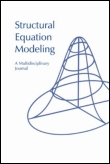# Co-Creation

Structural Equation Modeling

# On Estimating Finite Mixtures of Multivariate Regression and Simultaneous Equation Models - Structural Equation ModelingJedidi, Kamel, Venkatram Ramaswamy, Wayne S. DeSarbo, and Michel Wedel (1996), “On Estimating Finite Mixtures of Multivariate Regression and Simultaneous Equation Models,” Structural Equation Modeling, 3 (3), 266-289.